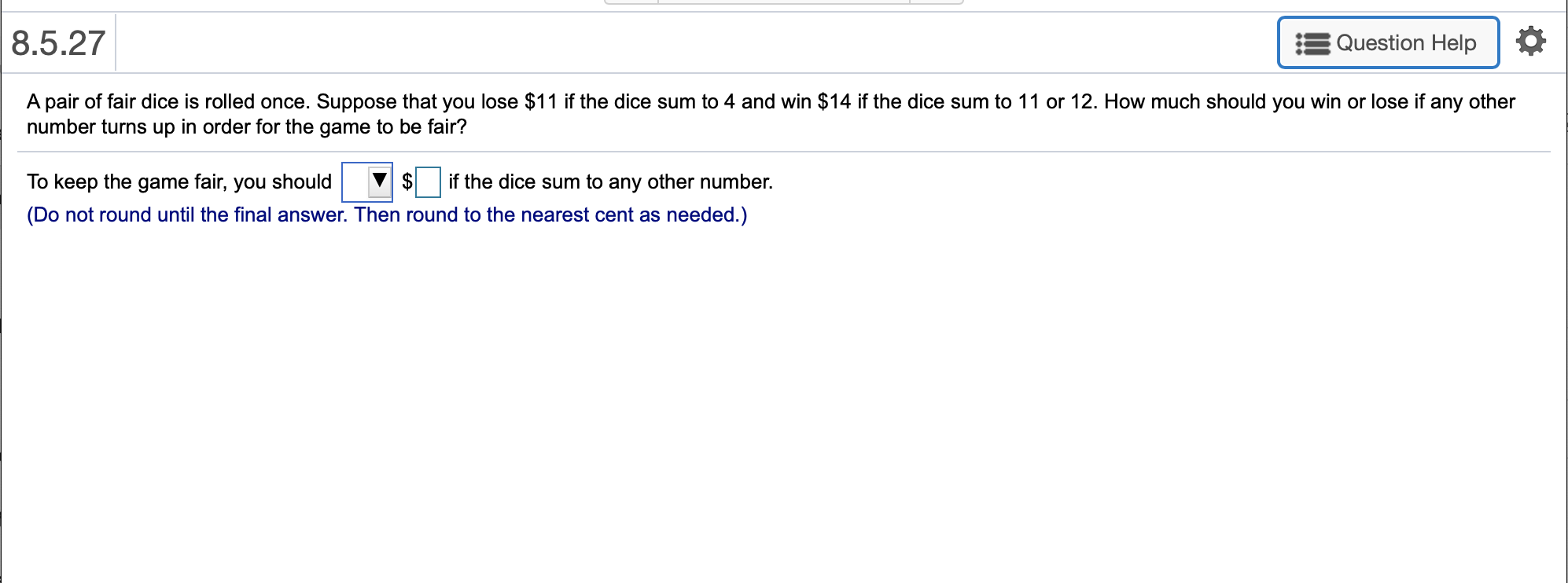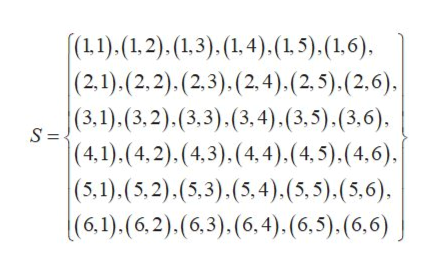# 8.5.27Question HelpA pair of fair dice is rolled once. Suppose that you lose \$11 if the dice sum to 4 and win \$14 if the dice sum to 11 or 12. How much should you win or lose if any othernumber turns up in order for the game to be fair?\$To keep the game fair, you shouldif the dice sum to any other number.(Do not round until the final answer. Then round to the nearest cent as needed.)

Question
12 viewshelp_outlineImage Transcriptionclose8.5.27 Question Help A pair of fair dice is rolled once. Suppose that you lose \$11 if the dice sum to 4 and win \$14 if the dice sum to 11 or 12. How much should you win or lose if any other number turns up in order for the game to be fair? \$ To keep the game fair, you should if the dice sum to any other number. (Do not round until the final answer. Then round to the nearest cent as needed.) fullscreen
check_circle

Step 1

The sample space of the 36 possible outcomes for a pair of fair dice is,help_outlineImage Transcriptionclose(11).(1,2).(1,3). (1,4). (1,5). (1,6) (2,1).(2,2).(2,3).(2,4) (2,5). (2,6). (3,1).(3,2).(3,3).(3,4).(3,5).(3,6) S = |(4,1).(4,2).(4,3). (4,4). (4, 5). (4,6) | (5,1).(5,2).(5,3).(5,4). (5,5).(5,6) (6,1). (6,2).(6,3).(6,4). (6,5). (6,6) fullscreen
Step 2

The pairs that give a sum of 4 are (1,3), (2,2) and (3,1).

So, the probability of getting a sum of 4 is,

P(4) = 3/36

And the pairs that give a sum of 11 or 12 are (5,6), (6, 5) and (6,6).

So, the probabili...

### Want to see the full answer?

See Solution

#### Want to see this answer and more?

Solutions are written by subject experts who are available 24/7. Questions are typically answered within 1 hour.*

See Solution
*Response times may vary by subject and question.
Tagged in

### Random Processes